Next: Vector magnitude Up: Motion in 3 dimensions Previous: Vector displacement

Suppose that the vector displacement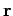of some pointfrom the originis specified as follows: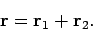(31)

Figure 12 illustrates how this expression is interpreted diagrammatically: in order to get from pointto point, we first move from pointto pointalong vector, and we then move from pointto pointalong vector. The net result is the same as if we had moved from pointdirectly to pointalong vector. Vectoris termed the resultant of adding vectorsand.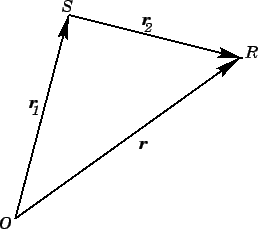Note that we have two ways of specifying the vector displacement of pointfrom the origin: we can either writeor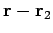. The expressionis interpreted as follows: starting at the origin, move along vectorin the direction of the arrow, then move along vectorin the opposite direction to the arrow. In other words, a minus sign in front of a vector indicates that we should move along that vector in the opposite direction to its arrow.

Suppose that the components of vectorsandare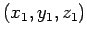and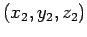, respectively. As is easily demonstrated, the components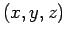of the resultant vector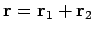are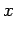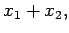(32)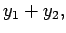(33)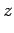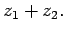(34)

In other words, the components of the sum of two vectors are simply the algebraic sums of the components of the individual vectors.Next: Vector magnitude Up: Motion in 3 dimensions Previous: Vector displacement
Richard Fitzpatrick 2006-02-02